# Closest Packing of Spheres How do spheres atoms

• Slides: 10Closest Packing of Spheres How do spheres (atoms) pack to best fill space? ? The concept of closest packing is important for understanding many solid structures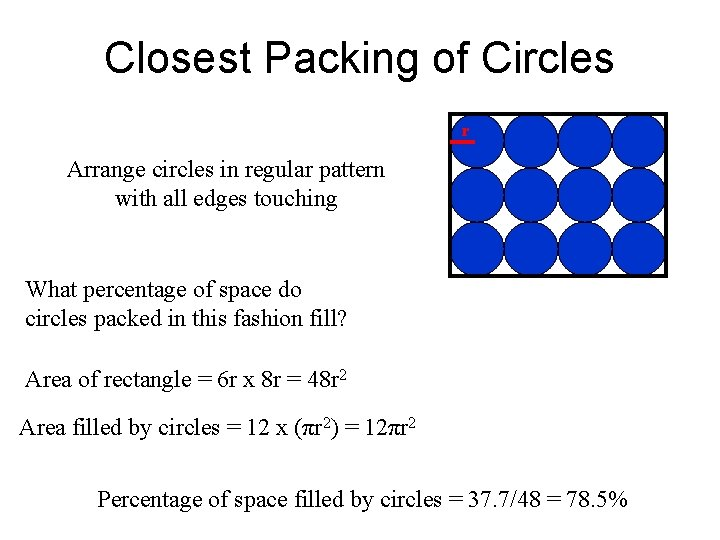Closest Packing of Circles r Arrange circles in regular pattern with all edges touching What percentage of space do circles packed in this fashion fill? Area of rectangle = 6 r x 8 r = 48 r 2 Area filled by circles = 12 x (πr 2) = 12πr 2 Percentage of space filled by circles = 37. 7/48 = 78. 5%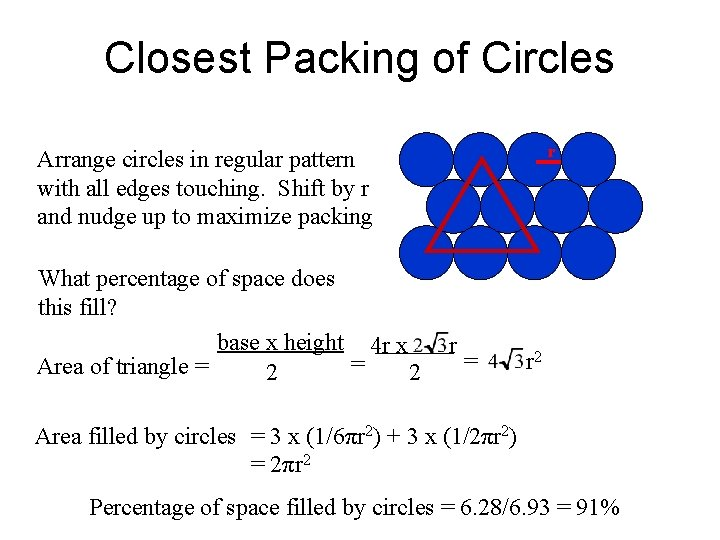Closest Packing of Circles r Arrange circles in regular pattern with all edges touching. Shift by r and nudge up to maximize packing What percentage of space does this fill? base x height 4 r x = Area of triangle = 2 2 r = r 2 Area filled by circles = 3 x (1/6πr 2) + 3 x (1/2πr 2) = 2πr 2 Percentage of space filled by circles = 6. 28/6. 93 = 91%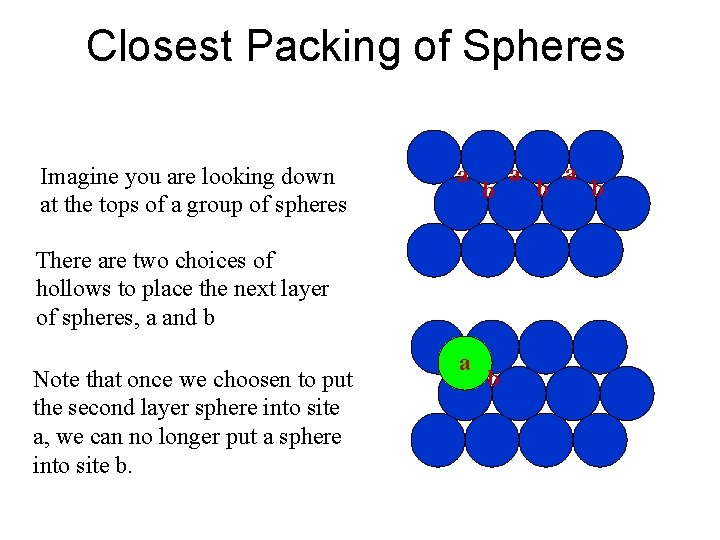Closest Packing of Spheres Imagine you are looking down at the tops of a group of spheres a b There are two choices of hollows to place the next layer of spheres, a and b Note that once we choosen to put the second layer sphere into site a, we can no longer put a sphere into site b. a b a bClosest Packing of Spheres Imagine you are looking down at the tops of two layers of spheres y x Note, we have two type of sites for the third layer, x and y. Site x is located above layer one sphere Site y is located above layer one hole Note, once again we can only fill x or y sites, not both, with new spheres y x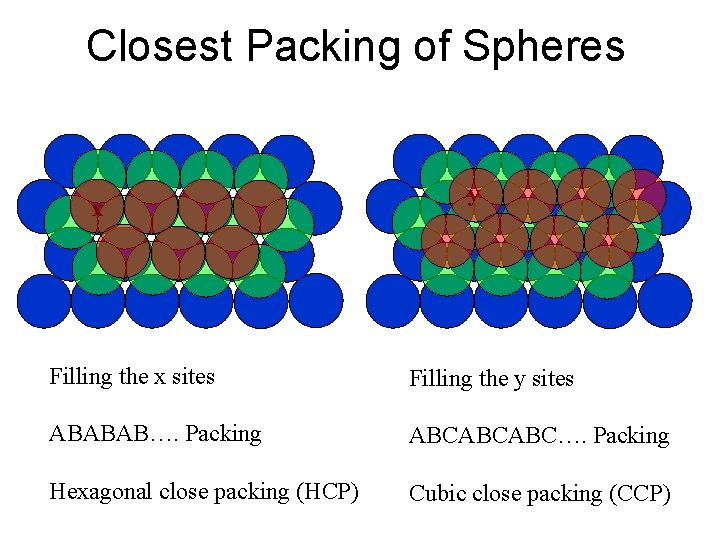Closest Packing of Spheres x y Filling the x sites Filling the y sites ABABAB…. Packing ABCABCABC…. Packing Hexagonal close packing (HCP) Cubic close packing (CCP)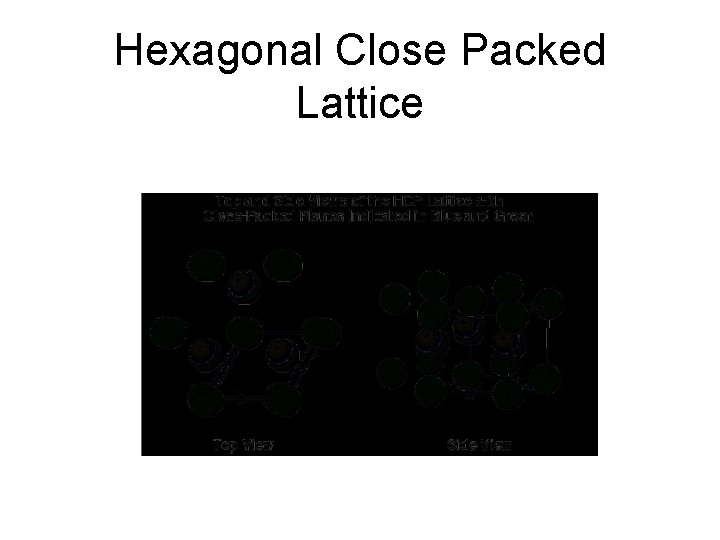Hexagonal Close Packed Lattice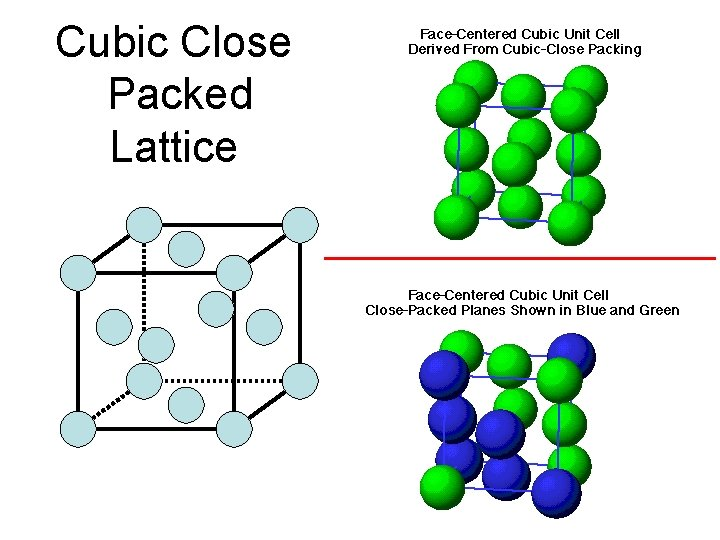Cubic Close Packed Lattice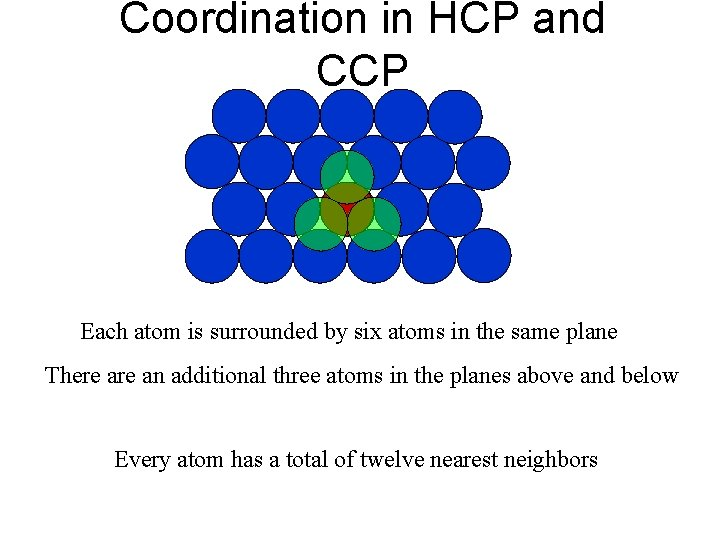Coordination in HCP and CCP Each atom is surrounded by six atoms in the same plane There an additional three atoms in the planes above and below Every atom has a total of twelve nearest neighborsPercent of Volume Filled by Close-Packed Spheres Calculate for FCC cell: Let radius of spheres = r • cell diagonal = 4 r • cell edge = r Volume of spheres = 4 x (4/3πr 3) Volume of cube = 4 atoms in cell volume of spheres = 16. 76/22. 63 = 0. 74 volume of cube Percent volume occupied = 74%